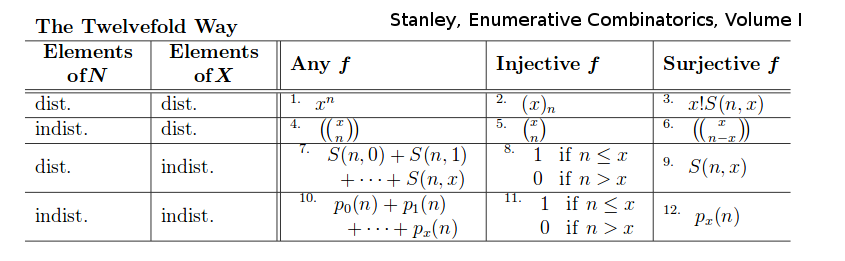# Book: Objects

I want to list and generate the basic combinatorial objects.

Stanley Enumerative Combinatorics

• Volume I
• Volume II: Table of Contents
• The Twelvefold Way
• Permutations
• Sieve methods - Inclusion Exclusion
• Partially ordered sets
• Rational generating functions
• Trees and the Composition of Generating Functions
• Algebraic, D-Finite, and Noncommutative Generating Functions
• Symmetric functions

The Twelvefold Way f:N->X two sets

• f is arbitrary (no restriction)
• f is injective (one-to-one)
• f is surjective (onto)

And regarding the elements of N and X as "distinguishable" or "indistinguishable".{$(x)_{n}=x(x-1)(x-2)...(x-n+1)$}

{$S(n,k)$} is the number of partitions of an n-set into k-blocks. It is called a Stirling number of the second kind.

Parsiųstas iš http://www.ms.lt/sodas/Book/Objects
Puslapis paskutinį kartą pakeistas 2018 sausio 07 d., 18:57